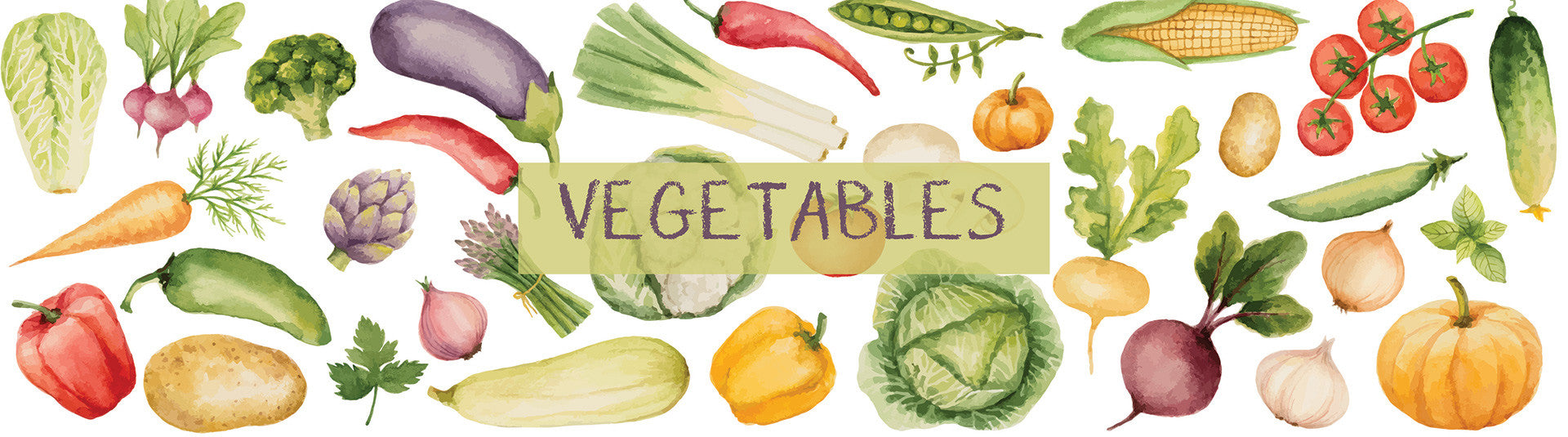Alocasia tubers (Arbi)
Unit
Yogis Pass Price: Rs. 36.00
Rs. 40.00
Baby Corn
Unit
Yogis Pass Price: Rs. 81.00
Rs. 90.00
Banana - Raw
Unit
Yogis Pass Price: Rs. 28.13
Rs. 31.25
Basil
Unit
Yogis Pass Price: Rs. 63.00
Rs. 70.00
Beans - French/String
Unit
Yogis Pass Price: Rs. 49.50
Rs. 55.00
Beetroot
Unit
Yogis Pass Price: Rs. 36.00
Rs. 40.00
Bitter Gourd (Karela)
Unit
Yogis Pass Price: Rs. 42.75
Rs. 47.50
Bottle Gourd (Ghia)
Unit
Yogis Pass Price: Rs. 58.50
Rs. 65.00
Brinjal Big
Unit
Yogis Pass Price: Rs. 31.50
Rs. 35.00
Brinjal Small
Unit
Yogis Pass Price: Rs. 29.25
Rs. 32.50
Broccoli
Unit
Yogis Pass Price: Rs. 179.64
Rs. 199.60
Cabbage
Unit
Yogis Pass Price: Rs. 63.00
Rs. 70.00
Capsicum
Unit
Yogis Pass Price: Rs. 42.75
Rs. 47.50
Carrot (Red)
Unit
Yogis Pass Price: Rs. 44.77
Rs. 49.75
Carrot- Orange
Unit
Yogis Pass Price: Rs. 44.77
Rs. 49.75
Cauliflower
Unit
Yogis Pass Price: Rs. 89.55
Rs. 99.50
Celery
Unit
Yogis Pass Price: Rs. 129.38
Rs. 143.75
Cherry Tomatoes
Unit
Yogis Pass Price: Rs. 123.75
Rs. 137.50
Choliya (Green Chana)
Unit
Yogis Pass Price: Rs. 269.55
Rs. 299.50
Coriander
Unit
Yogis Pass Price: Rs. 31.50
Rs. 35.00
Corn on the Cob/Sweet Corn
Unit
Yogis Pass Price: Rs. 67.50
Rs. 75.00
Cucumber
Unit
Yogis Pass Price: Rs. 29.25
Rs. 32.50
Cucumber - English/Seedless
Unit
Yogis Pass Price: Rs. 42.75
Rs. 47.50
Fenugreek leaves (Methi)
Unit
Yogis Pass Price: Rs. 51.75
Rs. 57.50
Garlic
Unit
Yogis Pass Price: Rs. 32.40
Rs. 36.00
Ginger
Unit
Yogis Pass Price: Rs. 30.60
Rs. 34.00
Green Chillies
Unit
Yogis Pass Price: Rs. 8.96
Rs. 9.95
Green Lettuce
Unit
Yogis Pass Price: Rs. 146.25
Rs. 162.50
Ice Berg Lettuce
Unit
Yogis Pass Price: Rs. 269.55
Rs. 299.50
Indian Gooseberry (Amla)
Unit
Yogis Pass Price: Rs. 78.75
Rs. 87.50
Jackfruit Raw - Cut Pieces
Unit
Yogis Pass Price: Rs. 146.25
Rs. 162.50
Unit
Yogis Pass Price: Rs. 44.77
Rs. 49.75
Leek
Unit
Yogis Pass Price: Rs. 112.50
Rs. 125.00
Lemon
Unit
Yogis Pass Price: Rs. 31.50
Rs. 35.00
Local Greens (Bathua)
Unit
Yogis Pass Price: Rs. 56.25
Rs. 62.50
Lotus Stem (Kamal Kakdi)
Unit
Yogis Pass Price: Rs. 67.50
Rs. 75.00
Mint
Unit
Yogis Pass Price: Rs. 10.00
Rs. 12.00
Mushroom
Unit
Yogis Pass Price: Rs. 108.00
Rs. 120.00
Mustard Greens (Sarson)
Unit
Yogis Pass Price: Rs. 67.50
Rs. 75.00
Onion
Unit
Yogis Pass Price: Rs. 49.50
Rs. 55.00
Parsley
Unit
Yogis Pass Price: Rs. 67.50
Rs. 75.00
Peas
Unit
Yogis Pass Price: Rs. 73.13
Rs. 81.25
Pepper Red
Unit
Yogis Pass Price: Rs. 126.00
Rs. 140.00
Pepper Yellow
Unit
Yogis Pass Price: Rs. 126.00
Rs. 140.00
Potato
Unit
Yogis Pass Price: Rs. 31.50
Rs. 35.00
Unit
Yogis Pass Price: Rs. 40.50
Rs. 45.00
Raw Papaya
Unit
Yogis Pass Price: Rs. 135.00
Rs. 150.00
Raw Turmeric
Unit
Yogis Pass Price: Rs. 22.50
Rs. 25.00
Sponge Gourd (Tori)
Unit
Yogis Pass Price: Rs. 50.63
Rs. 56.25
Spring Onion
Unit
Yogis Pass Price: Rs. 56.25
Rs. 62.50
Unit
Yogis Pass Price: Rs. 89.55
Rs. 99.50
Tomato - Desi
Unit
Yogis Pass Price: Rs. 63.00
Rs. 70.00
Tomato - Hybrid
Unit
Yogis Pass Price: Rs. 63.00
Rs. 70.00
Zucchini - Green
Unit
Yogis Pass Price: Rs. 84.38
Rs. 93.75
Zucchini - Yellow
Unit
Yogis Pass Price: Rs. 89.78
Rs. 99.75
My Box